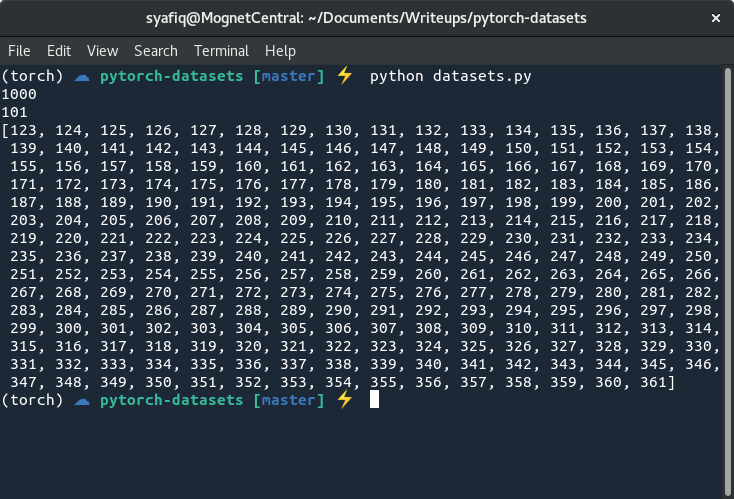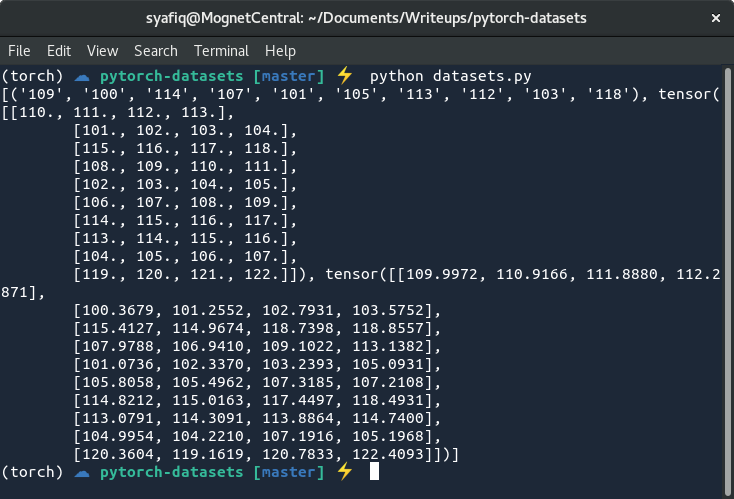# 在PyTorch中构建高效的自定义数据集PyTorch 最近已经出现在我的圈子里，尽管对Keras和TensorFlow感到满意，但我还是不得不尝试一下。令人惊讶的是，我发现它非常令人耳目一新，非常讨人喜欢，尤其是PyTorch 提供了一个Pythonic API、一个更为固执己见的编程模式和一组很好的内置实用程序函数。我特别喜欢的一项功能是能够轻松地创建一个自定义的Dataset对象，然后可以与内置的DataLoader一起在训练模型时提供数据。

## Dataset类的基础知识

Pythorch允许您自由地对“Dataset”类执行任何操作，只要您重写两个子类函数：

-返回数据集大小的函数，以及

-函数的函数从给定索引的数据集中返回一个样本。

PyTorch使您可以自由地对Dataset类执行任何操作，只要您重写改类中的两个函数即可：

• __len__ 函数：返回数据集大小
• __getitem__ 函数：返回对应索引的数据集中的样本

### 一个简单示例

from torch.utils.data import Dataset

class NumbersDataset(Dataset):
def __init__(self):
self.samples = list(range(1, 1001))

def __len__(self):
return len(self.samples)

def __getitem__(self, idx):
return self.samples[idx]

if __name__ == '__main__':
dataset = NumbersDataset()
print(len(dataset))
print(dataset)
print(dataset[122:361])### 扩展数据集

from torch.utils.data import Dataset

class NumbersDataset(Dataset):
def __init__(self, low, high):
self.samples = list(range(low, high))

def __len__(self):
return len(self.samples)

def __getitem__(self, idx):
return self.samples[idx]

if __name__ == '__main__':
dataset = NumbersDataset(2821, 8295)
print(len(dataset))
print(dataset)
print(dataset[122:361])### 从文件读取数据

• 创建一个全新的使用Python I/O和一些静态文件的Dataset
• 收集TES角色名称（我的网站上有可用的数据集），这些角色名称分为种族文件夹和性别文件，以填充samples列表
• 通过在samples列表中存储一个元组而不只是名称本身来跟踪每个名称的种族和性别。

TES名称数据集具有以下目录结构：

.
|-- Altmer/
|   |-- Female
|   -- Male
|-- Argonian/
|   |-- Female
|   -- Male
... (truncated for brevity)(为了简洁，这里进行省略)
-- Redguard/
|-- Female
-- Male

import os
from torch.utils.data import Dataset

class TESNamesDataset(Dataset):
def __init__(self, data_root):
self.samples = []

for race in os.listdir(data_root):
race_folder = os.path.join(data_root, race)

for gender in os.listdir(race_folder):
gender_filepath = os.path.join(race_folder, gender)

with open(gender_filepath, 'r') as gender_file:
for name in gender_file.read().splitlines():
self.samples.append((race, gender, name))

def __len__(self):
return len(self.samples)

def __getitem__(self, idx):
return self.samples[idx]

if __name__ == '__main__':
dataset = TESNamesDataset('/home/syafiq/Data/tes-names/')
print(len(dataset))
print(dataset)

# 将main函数改成下面这样：
if __name__ == '__main__':
dataset = TESNamesDataset('/home/syafiq/Data/tes-names/')
print(dataset[10:60])# 将main函数改成下面这样：
if __name__ == '__main__':
from torch.utils.data import DataLoader
dataset = TESNamesDataset('/home/syafiq/Data/tes-names/')
for i, batch in enumerate(dataloader):
print(i, batch)### 张量(tensor)和其他类型

from torch.utils.data import Dataset
import torch

class NumbersDataset(Dataset):
def __init__(self, low, high):
self.samples = list(range(low, high))

def __len__(self):
return len(self.samples)

def __getitem__(self, idx):
n = self.samples[idx]
successors = torch.arange(4).float() + n + 1
noisy = torch.randn(4) + successors
return n, successors, noisy

if __name__ == '__main__':
from torch.utils.data import DataLoader

dataset = NumbersDataset(100, 120)
print(next(iter(dataloader)))## 完成TES数据集的代码

• 更新构造函数以包含字符集
• 创建一个内部函数来初始化数据集
• 创建一个将标量转换为独热(one-hot)张量的工具函数
• 创建一个工具函数，该函数将样本数据转换为种族，性别和名称的三个独热(one-hot)张量的集合。

import os
from sklearn.preprocessing import LabelEncoder
from torch.utils.data import Dataset
import torch

class TESNamesDataset(Dataset):
def __init__(self, data_root, charset):
self.data_root = data_root
self.charset = charset
self.samples = []
self.race_codec = LabelEncoder()
self.gender_codec = LabelEncoder()
self.char_codec = LabelEncoder()
self._init_dataset()

def __len__(self):
return len(self.samples)

def __getitem__(self, idx):
race, gender, name = self.samples[idx]
return self.one_hot_sample(race, gender, name)

def _init_dataset(self):
races = set()
genders = set()

for race in os.listdir(self.data_root):
race_folder = os.path.join(self.data_root, race)

for gender in os.listdir(race_folder):
gender_filepath = os.path.join(race_folder, gender)

with open(gender_filepath, 'r') as gender_file:
for name in gender_file.read().splitlines():
self.samples.append((race, gender, name))

self.race_codec.fit(list(races))
self.gender_codec.fit(list(genders))
self.char_codec.fit(list(self.charset))

def to_one_hot(self, codec, values):
value_idxs = codec.transform(values)

def one_hot_sample(self, race, gender, name):
t_race = self.to_one_hot(self.race_codec, [race])
t_gender = self.to_one_hot(self.gender_codec, [gender])
t_name = self.to_one_hot(self.char_codec, list(name))
return t_race, t_gender, t_name

if __name__ == '__main__':
import string

data_root = '/home/syafiq/Data/tes-names/'
charset = string.ascii_letters + "-' "
dataset = TESNamesDataset(data_root, charset)
print(len(dataset))
print(dataset)

### 工具函数## ……但我们还没有### 可能的解决方案

• 将批处理(batch)大小设置为1，这样您就永远不会遇到错误。如果批处理大小为1，则单个张量不会与（可能）不同长度的其他任何张量堆叠在一起。但是，这种方法在进行训练时会受到影响，因为神经网络在单批次(batch)的梯度下降时收敛将非常慢。另一方面，当批次大小不重要时，这对于快速测试时，数据加载或沙盒测试很有用。
• 通过使用空字符填充或截断名称来获得固定的长度。截短长的名称或用空字符来填充短的名称可以使所有名称格式正确，并具有相同的输出张量大小，从而可以进行批处理。不利的一面是，根据任务的不同，空字符可能是有害的，因为它不能代表原始数据。

...
def __init__(self, data_root, charset, length):
self.data_root = data_root
self.charset = charset + '\0'
self.length = length
...
with open(gender_filepath, 'r') as gender_file:
for name in gender_file.read().splitlines():
if len(name) < self.length:
name += '\0' * (self.length - len(name))
else:
name = name[:self.length-1] + '\0'
self.samples.append((race, gender, name))
...• 堆叠种族张量，独热编码形式表示该张量是十个种族中的某一个种族
• 堆叠性别张量，独热编码形式表示数据集中存在两种性别中的某一种性别
• 堆叠名称张量，最后一个维度应该是charset的长度，第二个维度是名称长度（固定大小后），第一个维度是批(batch)大小。